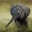Related Tags

c++

# How to find the smallest subarray whose sum is greater than xChayshta Singh

### Overview

The following article explains how to find the smallest subarray whose elements sum to a value greater than x.

### Algorithm

This problem can be solved in O(n) time using the two-pointers method. The idea is to maintain pointers that point to the first and last value of a subarray.

Initially, both left and right pointers (p and q) point at the first element. In every iteration, the right pointer q moves one step to the right. When the sum of the subarray between p and q is greater than x, a candidate solution is found. This subarray may also contain other such subarrays which sum to a value greater than x. They are obtained by moving p to the right. We start the next iteration either when p exceeds q, or the obtained subarray sum between p and q is less than or equal to x. The solution is the smallest-sized candidate solution found from all iterations.

The following image shows an example:

1 of 10

### Code

#include <iostream>
#include <vector>
#include <utility>
#include <numeric>

using namespace std;

//returns the first index and last index of smallest subarray
pair<int, int> findSmallestSubarray(vector<int> &vec, int x){
int p = 0;		//left pointer
int q = 0;		//right pointer
int sum = 0;
int bestp = -1, bestq = vec.size();		//saves result

for(q = 0; q < vec.size(); q++){
sum += vec[q];		//extends current subarray to the right
while(p <= q && sum > x){		//candidate solution found
int currSize = q - p + 1;
if(currSize < (bestq - bestp + 1)){		//save smallest subarray
bestp = p;
bestq = q;
}
sum -= vec[p++];	//reduces current subarray from the left
}
}

return make_pair(bestp, bestq);
}

void printVector(vector<int> &v);

int main(int argc, char const *argv[]){
vector<int> input{1, 5, 4, 10};
int x = 8;

auto p = findSmallestSubarray(input, x);
printVector(input);
cout << "x = " << x;
cout << "\nStart index: "<<p.first << ", End index: " << p.second;
cout << "\nSize of Subarray :" << (p.second - p.first + 1);
cout << "\nSum of Subarray : " << std::accumulate(input.begin() + p.first, input.begin() + p.second + 1, 0);

return 0;
}
Find the smallest subarray

### Time complexity

The time complexity of the above algorithm is O(n) where n is the size of the array.

### Explanation

• Line 16: The right pointer q moves one step to the right and updates the subarray sum.
• Lines 17–24: When the sum of the subarray is greater than x, we reduce the subarray from the left. To find the smallest subarray, compare the length of the subarray with sum greater than x to the saved best solution. The length of the subarray is found by q - p + 1.

RELATED TAGS

c++

CONTRIBUTORChayshta Singh
RELATED COURSES

View all Courses

Keep Exploring

Learn in-demand tech skills in half the time